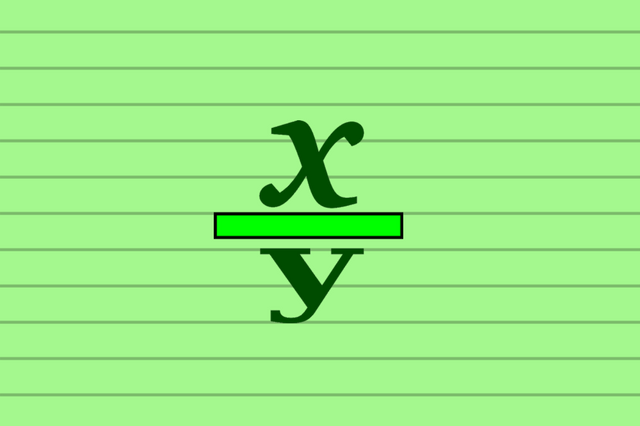Fractions

Year 1 Fractions Worksheets are available here.

Know about fractions for kids in year 1.
What is fraction?
A fraction is a numerical quantity that is not a whole number e.g. ½ etc.
In daily life fraction is a part or portion of something e.g. half portion of a pizza is equal to ½ of 1 pizza.
So numbers that are part of 1 are called fractions e.g. half of 1, quarter of 1, one third, one eighths, one hundredths and one millionths.
In mathematics these fractions are written
• Half of 1 =1/2. (It makes the half part of 1).
• Quarter of 1 =1/4. (It makes the 4th part of 1).

So
½ means 1 out of 2.
¼ means 1 out of 4.

Fun way to learn about Wholes, Half and Quarter.

A common fraction consists of a number called Numerator which is displayed above the line and non-zero number called Denominator displayed below the line. Both numerator and denominator should not be common.Fun way to learn about numerator and denominator is to click here.

For example, in the fraction 1/4, the numerator is 1, and the denominator is 4.
Here numerator 1 tell us that fraction represents 1  parts while denominator 4 tells us that 4 parts make up a whole.

In Year  1 pupils should be taught to recognise, find and name a half as one of two equal parts of an object, shape or quantity.

After learning the fractions You can click on Images of Worksheets to download Free.